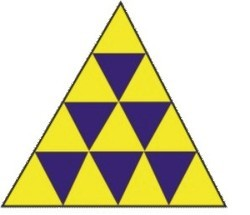0
<-- nev left start -->

# Ratio of Area of Similar TrianglesRatio of Area of Similar Triangles
Product Description

Ratio of Area of Similar Triangles

Ratio of Area of Similar Triangles : To verify the result that ratio of the areas of two similar triangles is equal to the ratio of the squares of there corresponding sides.